助力秋招第三弹：Java 集合框架体系详细梳理一、集合类

1，用于存储对象的容器。（容器本身就是一个对象，存在于堆内存中，里面存的是对象的地址）2，集合的长度是可变的。3，集合中不可以存储基本数据类型值。 （只能存对象）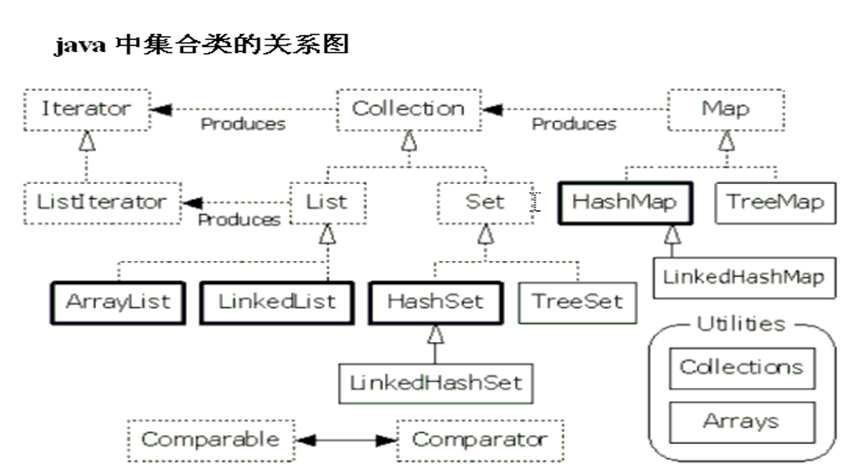二、 Collection 接口

Collection 子接口以及常用实现类

Collection 接口

• |--List 接口：有序(存入和取出的顺序一致),元素都有索引(角标)，元素可以重复。

• |--Vector:内部是数组数据结构，是同步的。增删，查询都很慢！100%延长（几乎不用了）

• |--ArrayList:内部是数组数据结构，是不同步的。替代了 Vector，查询的速度快，增删速度慢。50%延长。（查询时是从容器的第一个元素往后找，由于数组的内存空间是连续的，所以查询快；增删的话所有元素内存地址都要改变，所以增删慢。）

• |--Set 接口：无序，元素不能重复。Set 接口中的方法和 Collection 一致。

• |--HashSet: 内部数据结构是哈希表 ，是不同步的。

• |--TreeSet：内部数据结构是有序的二叉树，它的作用是提供有序的 Set 集合，是不同步的。

List 接口

Set 和 List 的区别

1. Set 接口实例存储的是无序的，不重复的数据。List 接口实例存储的是有序的，可以重复的元素 <最本质区别>

2. Set 检索效率低下，删除和插入效率高，插入和删除不会引起元素位置改变 。

3. List 和数组类似，可以动态增长，根据实际存储的数据的长度自动增长 List 的长度。查找元素效率高，插入删除效率低，因为会引起其他元素位置改变  。

ArryList 和 Vector 可变长度数组的原理

ArryList 是线程不安全的，Vector 是安全的

ArryList 存取对象的一个小例子

Person p1 = new Person("lisi1",21);                  ArrayList al = new ArrayList();        al.add(p1);        al.add(new Person("lisi2",22));        al.add(new Person("lisi3",23));        al.add(new Person("lisi4",24));                  Iterator it = al.iterator();        while(it.hasNext()){//          System.out.println(((Person) it.next()).getName()+"::"+((Person) it.next()).getAge());　　　　　　　　　　　　//错误方式：不能这样取，next()一次指针会移动一次，会输出“lisi1::22 lisi3::24”            // 正确方式：拿到一个Person对象，然后取属性。            Person p = (Person) it.next();            System.out.println(p.getName()+"--"+p.getAge());        }

HashSet 之覆盖 hashCode 方法和 equals 方法来保证元素唯一性

• ->如果对象的 hashCode 值不同，那么不用判断 equals 方法，就直接存储到哈希表中。

• ->如果对象的 hashCode 值相同，那么要再次判断对象的 equals 方法是否为 true:

import java.util.HashSet;import java.util.Iterator; class Person {     private String name;    private int age;     public Person(String name, int age) {        this.name = name;        this.age = age;    }     @Override    public int hashCode() {        // System.out.println(this+".......hashCode");        return name.hashCode() + age * 27; // 乘以一个任意数，防止加了年龄以后HashCode仍相同    }     @Override    public boolean equals(Object obj) {        // 健壮性判断        if (this == obj)            return true;        if (!(obj instanceof Person))            throw new ClassCastException("类型错误");        // System.out.println(this+"....equals....."+obj);         Person p = (Person) obj;        return this.name.equals(p.name) && this.age == p.age;    }     public String getName() {        return name;    }     public void setName(String name) {        this.name = name;    }     public int getAge() {        return age;    }     public void setAge(int age) {        this.age = age;    }     public String toString() {        return name + ":" + age;    }} public class HashSetTest {     public static void main(String[] args) {        HashSet hs = new HashSet();        /*         * HashSet集合数据结构是哈希表，所以存储元素的时候，         * 使用的元素的hashCode方法来确定位置，如果位置相同，在通过元素的equals来确定是否相同。         *         */        hs.add(new Person("lisi4", 24));        hs.add(new Person("lisi7", 27));        hs.add(new Person("lisi1", 21));        hs.add(new Person("lisi9", 29));        hs.add(new Person("lisi7", 27));         Iterator it = hs.iterator();        while (it.hasNext()) {            Person p = (Person) it.next();            System.out.println(p);        }    }}

lisi1:21lisi9:29lisi4:24lisi7:27

TreeSet 之判断元素唯一性的两种方式（如何排序）

TreeSet 默认判断元素唯一性的方式

import java.util.Iterator;import java.util.TreeSet; class Person implements Comparable {     public String name;    public int age;     public Person() {        super();     }     public Person(String name, int age) {        super();        this.name = name;        this.age = age;    }     public String toString() {        return name + ":" + age;    }     @Override    public int compareTo(Object o) {        Person p = (Person) o;         /* 敲黑板划重点，代码简洁方式 */        int temp = this.age - p.age;        return temp == 0 ? this.name.compareTo(p.name) : temp;         // 上面这两句相当于底下这一段的简洁形式        // if (this.age > p.age)        // return 1;        // if (this.age < p.age)        // return -1;        // else {        // return this.name.compareTo(p.name);        // }    }     public static void main(String[] args) {        TreeSet<Person> ts = new TreeSet<Person>();        ts.add(new Person("zhangsan", 22));        ts.add(new Person("lisi", 27));        ts.add(new Person("wangermazi", 21));        ts.add(new Person("zhaosi", 25));         Iterator it = ts.iterator();        while (it.hasNext()) {            Person person = (Person) it.next();            System.out.println(person.toString());        }    }}

wangermazi:21zhangsan:22zhaosi:25lisi:27

step1-新建比较器类 ComparedByName.java，覆盖 compare 方法：

import java.util.Comparator; public class ComparedByName implements Comparator {     @Override    public int compare(Object o1, Object o2) {        // TODO Auto-generated method stub        Person p1 = (Person) o1;        Person p2 = (Person) o2;        int temp = p1.name.compareTo(p2.name);        return temp == 0 ? p1.age - p2.age : temp;    }}

step2-将比较器类类对象作为参数传递给 TreeSet 集合的构造函数：

import java.util.Iterator;import java.util.TreeSet; class Person implements Comparable {     public String name;    public int age;     public Person() {        super();     }     public Person(String name, int age) {        super();        this.name = name;        this.age = age;    }     public String toString() {        return name + ":" + age;    }     @Override    public int compareTo(Object o) {        Person p = (Person) o;         /* 敲黑板划重点，代码简洁方式 */        int temp = this.age - p.age;        return temp == 0 ? this.name.compareTo(p.name) : temp;         // 上面这两句相当于底下这一段的简洁形式        // if (this.age > p.age)        // return 1;        // if (this.age < p.age)        // return -1;        // else {        // return this.name.compareTo(p.name);        // }    }     public static void main(String[] args) {        TreeSet<Person> ts = new TreeSet<Person>(new ComparedByName());        ts.add(new Person("zhangsan", 22));        ts.add(new Person("lisi", 27));        ts.add(new Person("wangermazi", 21));        ts.add(new Person("zhaosi", 25));         Iterator it = ts.iterator();        while (it.hasNext()) {            Person person = (Person) it.next();            System.out.println(person.toString());        }    }}

lisi:27wangermazi:21zhangsan:22zhaosi:25

三、Iterator 接口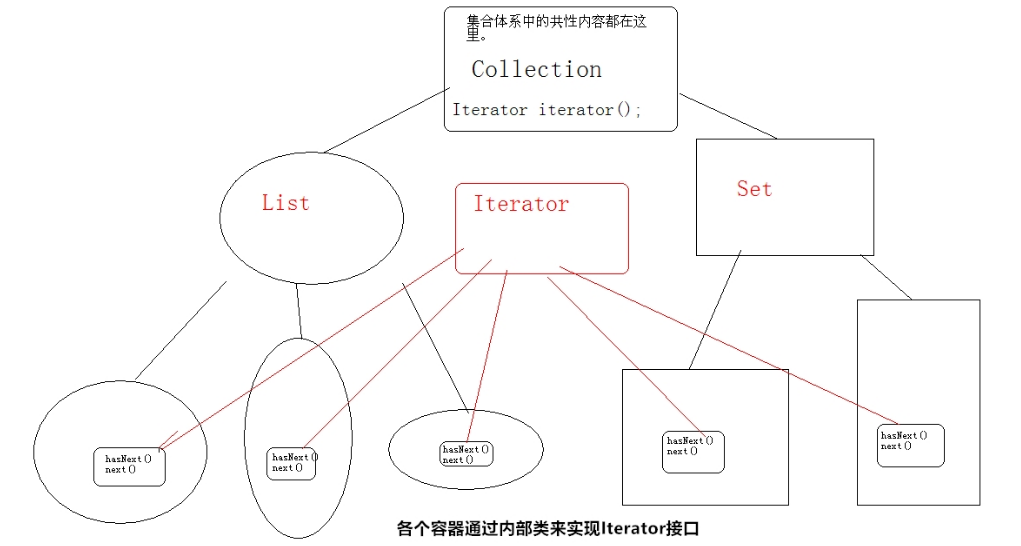Iterator 的一个子接口

|--ListIterator 接口（列表迭代器）

Iterator it = list.iterator();        while(it.hasNext()){                         Object obj = it.next();//java.util.ConcurrentModificationException                            //在使用迭代器的过程中使用集合中的方法add()操作元素，出现异常。                        //可以使用Iterator接口的子接口ListIterator来完成在迭代中对元素进行更多的操作。            　　　　　　　　            if(obj.equals("abc2")){                list.add("abc9");            }            else                System.out.println("next:"+obj);        }        System.out.println(list);

public static void main(String[] args) {         List list = new ArrayList();               list.add("abc1");        list.add("abc2");        list.add("abc3");                 System.out.println("list:"+list);        ListIterator it = list.listIterator();//获取列表迭代器对象        //它可以实现在迭代过程中完成对元素的增删改查。        //注意：只有list集合具备该迭代功能.                         while(it.hasNext()){                       Object obj = it.next();                       if(obj.equals("abc2")){                it.add("abc9"); //ListIterator提供了add方法            }        }

四、Map 接口

Map 接口与 Set 类似，可以对照着来学，比如比较器在 TreeMap 中也适用。

Map：    一次添加一对元素，Collection 一次添加一个元素。

Map 也称为双列集合，Collection 集合也称为单列集合。

1，添加

• value put(key,value):返回前一个和 key 关联的值，如果没有返回 null.

2，删除

• void clear():清空 map 集合。

• value remove(key):根据指定的 key 翻出这个键值对。

3，判断

• boolean containsKey(key):是否包含该 key

• boolean containsValue(value):是否包含该 value

• boolean isEmpty();是否为空

4，获取

value get(key):通过键获取值，如果没有该键返回 null。当然，可以通过是否返回 null，来判断是否包含指定键。

int size(): 获取键值对的个数。

Map 常用的子类：（HashMap 与 Hashtable 的区别，面试常问）

• |--Hashtable :内部结构是哈希表，是同步的。不允许 null 作为键，null 作为值。

• |--Properties：用来存储键值对型的配置文件的信息，可以和 IO 技术相结合。

• |--HashMap : 内部结构是哈希表，不是同步的。允许 null 作为键，null 作为值。

• |--TreeMap : 内部结构是二叉树，不是同步的。可以对 Map 集合中的键进行排序。

Map 的迭代方法：

Map 本身没有迭代器。

public class MapDemo {     public static void main(String[] args) {                 Map<Integer,String> map = new HashMap<Integer,String>();        method_2(map);    }         public static void method_2(Map<Integer,String> map){                 map.put(8,"zhaoliu");        map.put(2,"zhaoliu");        map.put(7,"xiaoqiang");        map.put(6,"wangcai");                         Collection<String> values = map.values();                 Iterator<String> it2 = values.iterator();        while(it2.hasNext()){            System.out.println(it2.next());        }        }}

Set<Integer> keySet = map.keySet();Iterator<Integer> it = keySet.iterator(); while(it.hasNext()){    Integer key = it.next();    String value = map.get(key);    System.out.println(key+":"+value);     }

Set<Map.Entry<Integer, String>> entrySet = map.entrySet(); Iterator<Map.Entry<Integer, String>> it = entrySet.iterator(); while(it.hasNext()){    Map.Entry<Integer, String> me = it.next();    Integer key = me.getKey();    String value = me.getValue();    System.out.println(key+":"+value);         }

for (Map.Entry<String, String> entry : map.entrySet()) {       System.out.println("key= " + entry.getKey() + " and value= " + entry.getValue());      }

map 中比较器的用法（百度面试题）

1 public class HashMapTest { 2     // 将内部内修改为静态，直接可以在main函数中创建内部类实例 3     private static class ValueComparator implements Comparator<Map.Entry<Character, String>> { 4         @Override 5         public int compare(Map.Entry<Character, String> entryA, Map.Entry<Character, String> entryB) { 6             // 复写的方法是compare，String类的方法是compareTo，不要记混。 7             return entryA.getValue().compareTo(entryB.getValue()); 8         } 9     }10 11     public static void main(String[] args) {12         Map<Character, String> map = new HashMap<>();13         map.put('c', "3");14         map.put('a', "5");15         map.put('b', "1");16         map.put('d', "2");17         System.out.println("Before Sort:");18         for (Map.Entry<Character, String> mapping : map.entrySet()) {19             System.out.println(mapping.getKey() + ":" + mapping.getValue());20         }21 22         List<Map.Entry<Character, String>> list = new ArrayList<>(map.entrySet());23         // 或者list.addAll(map.entrySet());24         ValueComparator vc = new ValueComparator();25         Collections.sort(list, vc);26 27         System.out.println("After Sort:");28         for (Map.Entry<Character, String> mapping : list) {29             System.out.println(mapping.getKey() + ":" + mapping.getValue());30         }31     }32 }

五、集合框架工具类 Collections 和 Arrays

Collections 是集合框架的工具类，里面的方法都是静态的。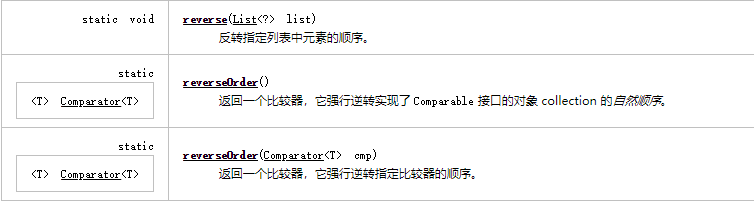import java.util.Comparator; public class ComparatorByLength implements Comparator<String> {     @Override    public int compare(String o1, String o2) {         int temp = o1.length() - o2.length();                 return temp==0?o1.compareTo(o2): temp;    }}

Demo：

public static void demo_3() {         // reverse实现原理        /*         * TreeSet<String> ts = new TreeSet<String>(new Comparator<String>() {             @Override            public int compare(String o1, String o2) {                 int temp = o2.compareTo(o1);                return temp;            }        });        */         TreeSet<String> treeset = new TreeSet<String>(new ComparatorByLength());        treeset.add("abc");        treeset.add("hahaha");        treeset.add("zzz");        treeset.add("aa");        treeset.add("cba");        System.out.println(treeset);         TreeSet<String> ts = new TreeSet<String>(Collections.reverseOrder(new ComparatorByLength()));//都是静态方法，直接类名调用         ts.add("abc");        ts.add("hahaha");        ts.add("zzz");        ts.add("aa");        ts.add("cba");         System.out.println("after reverse:\t" + ts);     }public static void main(String[] args) {　　　    　demo_3();　　}<em id="__mceDel" style="background-color: rgba(255, 255, 255, 1); font-family: "PingFang SC", "Helvetica Neue", Helvetica, Arial, sans-serif; font-size: 14px"><br></em>

public static void demo_2() {        List<String> list = new ArrayList<String>();         list.add("abcde");        list.add("cba");        list.add("aa");        list.add("zzz");        list.add("cba");        list.add("nbaa");        System.out.println(list);         Collections.sort(list);        System.out.println("after sort:\n" + list);         Collections.sort(list, Collections.reverseOrder());        System.out.println("after reverse sort:\n" + list);         int index = Collections.binarySearch(list, "cba");        System.out.println("index=" + index);         // 获取最大值。        String max = Collections.max(list, new ComparatorByLength());        System.out.println("maxLength=" + max);    }    public static void main(String[] args) {         demo_2();    }

[abcde, cba, aa, zzz, cba, nbaa]after sort:[aa, abcde, cba, cba, nbaa, zzz]after reverse sort:[zzz, nbaa, cba, cba, abcde, aa]index=2maxLength=abcde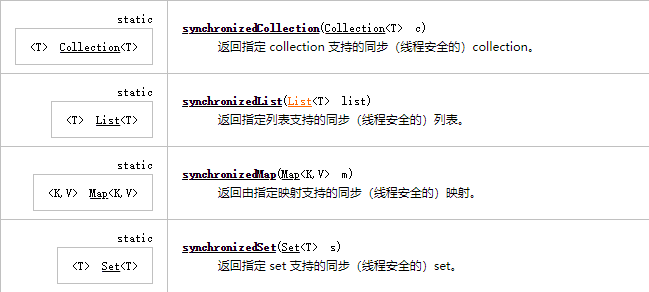List list = new ArrayList();// 非同步的list。     list=MyCollections.synList(list);// 返回一个同步的list. class MyCollections{                 /**         * 返回一个加锁的List         * */        public static  List synList(List list){                return new MyList(list);        }        // 内部类        private class MyList implements List{                 private List list;                 private static final Object lock = new Object();                 MyList(List list){             this.list = list;          }                 public boolean add(Object obj){            synchronized(lock)            {                return list.add(obj);            }        }                 public boolean remove(Object obj){            synchronized(lock)            {                return list.remove(obj);            }        }         }}

Demo：

public static void demo_1() {     String[] arr = { "abc", "haha", "xixi" };     List<String> list = Arrays.asList(arr);    boolean b1 = list.contains("xixi");    System.out.println("list contains:" + b1);    // list.add("hiahia");//引发UnsupportedOperationException     System.out.println(list);}

list contains:true[abc, haha, xixi]

Demo：

public static void demo_2() {    /*     * 如果数组中的元素是对象，那么转成集合时，直接将数组中的元素作为集合中的元素进行集合存储。     *     * 如果数组中的元素是基本类型数值，那么会将该数组作为集合中的元素进行存储。     *     */    int[] arr = { 31, 11, 51, 61 };     List<int[]> list = Arrays.asList(arr);     System.out.println(list);    System.out.println("数组的长度为：" + list.size());}

[[I@659e0bfd]数组的长度为：1

** 例 5：将数组转成集合，List.toArray()方法**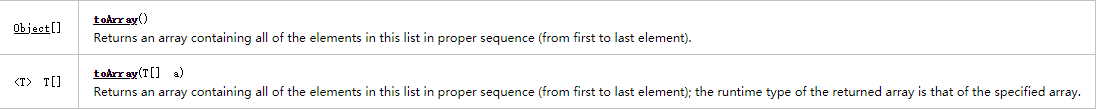Demo：

public class ToArray {    public static void main(String[] args) {         List<String> list = new ArrayList<String>();        list.add("abc1");        list.add("abc2");        list.add("abc3");                 String[] arr = list.toArray(new String[list.size()]);              System.out.println(Arrays.toString(arr));                  }}

for(类型 变量 ：Collection 集合**|数组) {

}

Demo：

public class ForEachDemo {     public static void main(String[] args) {                 // 遍历数组        int[] arr = { 3, 1, 5, 7, 4 };                 for (int i : arr) {            System.out.println(i);        }                 //遍历List        List<String> list = new ArrayList<String>();        list.add("abc1");        list.add("abc2");        list.add("abc3");         for (String s : list) {            System.out.println(s);        }         // 遍历map        // 可以使用高级for遍历map集合吗？不能直接用，但是将map转成单列的set，就可以用了。        Map<Integer, String> map = new HashMap<Integer, String>();        map.put(3, "zhagsan");        map.put(1, "wangyi");        map.put(7, "wagnwu");        map.put(4, "zhagsansan");         for (Integer key : map.keySet()) {            String value = map.get(key);            System.out.println(key + "::" + value);        }         for (Map.Entry<Integer, String> me : map.entrySet()) {            Integer key = me.getKey();            String value = me.getValue();             System.out.println(key + ":" + value);        }         // 老式的迭代器写法        Iterator<String> it = list.iterator();        while (it.hasNext()) {            System.out.println(it.next());        }    }}

end评论